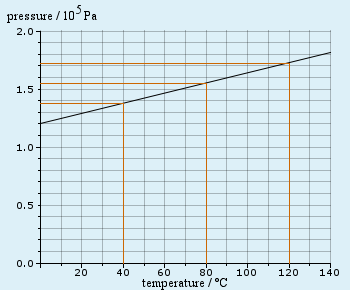# Finding the gradient: On a graph w/ pressure/105 Pa units, what is gradient?

#### Kyebas

##### New member
On a graph with pressure / 105 Pa units, with a y axis that goes up in 0.5s and an x axis that goes across in 20, you have a graph line stretching from 1.3 (0 on y axis) to 1.8 (140 on a y axis), what is the gradient to 3 significant figures?

I got 93.3 x 10 but I know I am wrong. Can someone explain where I went wrong?

#### Kyebas

##### New member
Finding a gradient help

On a graph with pressure / 105 Pa units, with a y axis that goes up in 0.5s and an x axis that goes across in 20, you have a graph line stretching from 1.3 (0 on y axis) to 1.8 (140 on a y axis), what is the gradient to 3 significant figures?

I got 93.3 x 10 but I know I am wrong. Can someone explaine where I went wrong?

#### stapel

##### Super Moderator
Staff member
On a graph with pressure / 105 Pa units...
Since "Pa" (or "Pascals") is a unit of pressure itself, I'm not understanding the "units" of "pressure per 105 Pascals"...? Also, are these the "units" for both of the axes?

...with a y axis that goes up in 0.5s...
Does this mean that the units on the y-axis are "seconds" and that the scale is marked off in half-second increments?

...and an x axis that goes across in 20...
What does "goes across in 20" mean? That the scale is marked off in twenty-unit increments (like twenty Pascals or something)? Or that the x-axis is twenty units wide? Or something else?

...you have a graph line stretching from 1.3 (0 on y axis) to 1.8 (140 on a y axis), what is the gradient to 3 significant figures?
Does this mean that the line has two marked points, being (x, y) = (1.3, 0) and (x, y) = (1.8, 140)?

I got 93.3 x 10 but I know I am wrong. Can someone explaine where I went wrong?
Sure! Show all of your work and reasoning, and we'll be glad to figure out where things started going sideways. Thank you!#### Kyebas

##### New member
Hi, Thanks! I think this will explain it better!

"The units on the graph below are shown as “pressure / 105 Pa”. This means that the value for pressure has been divided by 105 Pa, not simply by Pa, as you are used to. So a reading of 1 on the axis has a true value of 1 x 105 Pa. In an experiment carried out on a fixed amount of gas held at constant volume, the pressure (in units of Pa was measured as a function of the temperature (oC)Calculate the gradient of the resulting graph to two significant figures, expressing your answer in scientific notation."

I have calculated it as 93.3 x 105 but if it isn't completely wrong, I am sure I am missing a final step.

#### Attachments

• 20 KB Views: 2

#### j-astron

##### Junior Member
Hi, Thanks! I think this will explain it better!

"The units on the graph below are shown as “pressure / 105 Pa”. This means that the value for pressure has been divided by 105 Pa, not simply by Pa, as you are used to. So a reading of 1 on the axis has a true value of 1 x 105 Pa. In an experiment carried out on a fixed amount of gas held at constant volume, the pressure (in units of Pa was measured as a function of the temperature (oC)Calculate the gradient of the resulting graph to two significant figures, expressing your answer in scientific notation."

I have calculated it as 93.3 x 105 but if it isn't completely wrong, I am sure I am missing a final step.
As far as I can see, the y-axis goes from 1.2 to 1.8, not 1.3 to 1.8 as you stated in the original post.

The x-axis goes from 0 to 140 C

You have the change in y-value

You have the change in x-value

How do you compute the slope from that?

I will never understand why people don't show their steps. That's the only way for us to see where you went wrong. So, HOW did you arrive at an answer of 93.3? Show us your work.